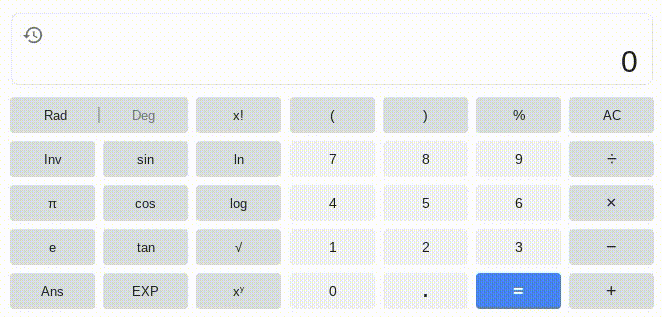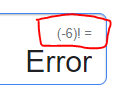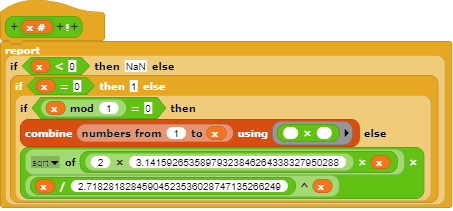# Factorial block from the BIGNUMS library cannot handle negative numbers

Apparently, trying to get the factorial of a negative number in Snap! slows down Snap! significantly.But in Google, it works fine.Sorry, either Google is wrong or you are misinterpreting how its calculator works. Since Google knows everything, I assume it's the latter, and when you enter −, 6, x! into the calculator it's actually giving you −(6!), not, as you think, (−6)!.

You are correct.That'll give you an answer, but the answer won't be the factorial of the negative integer, because it doesn't have one. Read the Wikipedia article.

Negative numbers that aren't integers can have factorials, if you use the gamma function to extend the domain. (Look up "gamma function.")

here is my version of the factorial block